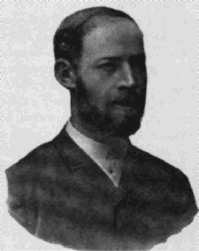EECS20N: Signals and Systems

# Frequencies in Hertz and Radians

A standard measure of frequency is Hertz, abbreviated Hz. It means "cycles per second." Here is a plot of one second of four sine waves of different frequencies:

If you were able to run applets, you would have a waveform here.

By convention, frequencies in Hertz are usually written using the symbol f. For example, the frequencies of the musical note A on the piano keyboard are

f1 = 55, f2 = 110, f3 = 220, f4 = 440, f5 = 880, f6 = 1760, f7 = 3520, f8 = 7040

A sinusoidal waveform with frequency f4 = 440 can be defined by

x(t) = cos(2π f4 t)

The factor 2π in the expression above is a nuisance. The argument to the cosine function has units of radians, so 2π has units of radians/cycle. Explicitly showing all the units (in square brackets),

To avoid having to keep track of the factor 2π everywhere, it is common to use the alternative units for frequency, radians per second. The symbol ω is commonly used to denote frequencies in radians per second. The relationship between Hertz and radians per second is simple,

π = 2π f

Thus, in radians per second, the frequencies of the musical note A on the piano keyboard are

π 1 = 2π × 55, ω 2 = 2π × 110, ω 3 = 2π × 220, ω 4 = 2π × 440,

π 5 = 2π × 880, ω 6 = 2π × 1760, ω 7 = 2π × 3520, ω 8 = 2π × 7040

# Discrete-time frequencies

When the domain of a signal is DiscreteTime = Integers, then the units of frequency are cycles/sample. Consider for example the discrete-time signal given by

nDiscreteTime, x(n) = cos(2π f n).

Suppose this represents an audio signal that is sampled at 8000 samples/second. Then to convert f to Hertz, just watch the units:

f [cycles/sample] × 8000 [samples/second] = 8000f [cycles/second].

The frequency could have been equally well given in units of radians/sample, as in

nDiscreteTime, x(n) = cos(ω n).

To convert ω to Hertz,

ω [radians/sample] × 8000 [samples/second] × (1/2π ) [cycles/radian] = (8000ω /2π ) [cycles/second].

# Ranges of frequencies

An extremely wide range of frequencies are used by electrical engineers. The following abbreviations are common:

• kHz - kilohertz, thousands of cycles per second.
• MHz - megahertz, millions of cycles per second.
• GHz - gigahertz, billions of cycles per second.

Audible sounds signals are in the range of about 20 Hz. to about 20 kHz. Sounds above this frequency are called "ultrasonic." Electromagnetic waves range from less than one hertz (used speculatively in seismology for earthquake prediction) through visible light near 1015 Hz. to cosmic ray radiation up to 1025 Hz. See uses of electromagnetic radiation in communication.

# Heinrich HertzHeinrich Rudolf Hertz (1847-1894), after whom the unit of frequency is named, was the first to transmit and receive radio waves. Both an experimentalist and a theoretician, Hertz rederived Maxwell's wave equations, which model the propagation of electromagnetic waves, casting them in the form used today. He eliminated Maxwell's unusual mechanical ideas about the role of the ether, which had impeded acceptance of his model. Between 1885 and 1889, while he was a professor of physics at Karlsruhe Polytechnic, he produced electromagnetic waves and measured their wavelength and velocity in the laboratory. He showed that the nature of their reflection and refraction was the same as those of light, supporting the theory that light waves are electromagnetic radiation obeying Maxwell's equations.

Source: Leonard S. Taylor's web page on historical figures in engineering.